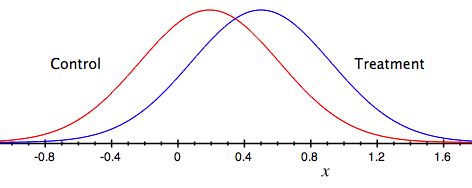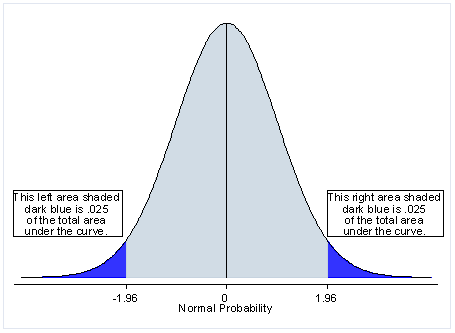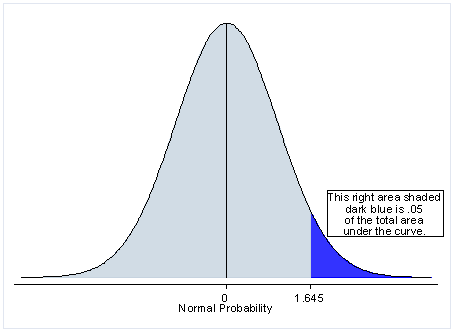## Statistical Analysis and A/B Testing

by Jesse Farmer on Tuesday, October 6, 2009

In this article we're going to talk about how hypothesis testing can tell you whether your A/B tests actually effect user behavior, or whether the variations you see are due to random chance.

First, if you haven't yet, read my previous introductory article on hypothesis testing. It explains the statistical principles behind hypothesis testing using the example of a biased coin. We're going to move quickly beyond that and dive right into A/B testing.

### Landing Page Conversion

You're testing a landing page that has a signup form. You want to test various layouts to try and maximize the percentage of people who sign up. This percentage is called the "conversion rate," i.e., the rate at which you convert visitors from passerbys to customers.

You have a four-way experiment with a control treatment and three experimental treatments. How you pick your treatments is a subject worth discussing in its own right, but they should try to move the big levers: copy, layout, and size.

For this experiment we'll just call the treatments control, A, B, and C. You can use your imagination.

### Fake Data

Your totally awesome Project X is attracting users. You've analyzed your sales pipeline and the point with the highest potential impact is the landing page. You want increase the landing page conversion rate by at least 20%.

You create an A/B test with four treatments: control, A, B, and C. Here is the data you collect:

Project X Landing Page
Treatment Visitors Treated Visitors Registered Conversion Rate
Control 182 35 19.23%
Treatment A 180 45 25.00%
Treatment B 189 28 14.81%
Treatment C 188 61 32.45%

From the data both treatments A and C show at least a 20% improvement in the landing page performance, which was our goal. You might declare Treatment C "good enough," choose it, and move on. But how do you know the variation isn't due to random chance? What if instead of 188 visitors treated we only had 10 visitors treated? Would you still be so confident?

As usual we're aiming for a 95% confidence interval.

Hypothesis testing is all about quantifying our confidence, so let's get to it.

### The Statistics

Remember, we need to start with a null hypothesis. In our case, the null hypothesis will be that the conversion rate of the control treatment is no less than the conversion rate of our experimental treatment. Mathematically

H_0: p - p_c \le 0

where pc is the conversion rate of the control and p is the conversion rate of one of our experiments.

The alternative hypothesis is therefore that the experimental page has a higher conversion rate. This is what we want to see and quantify.

The sampled conversion rates are all normally distributed random variables. It's just like the coin flip, except instead of heads or tails we have "converts" or "doesn't convert." Instead of seeing whether it deviates too far from a fixed percentage we want to measure whether it deviates too far from the control treatment.

Here's an example representation of the distribution of the control conversion rate and the treatment conversion rate.The peak of each curve is the conversion rate we measure, but there's some chance it is actually somewhere else on the curve. Moreover, what we're really interested in is the difference between the two conversion rates. If the difference is large enough we conclude that the treatment really did alter user behavior.

So, let's define a new random variable

X = p - p_c
then our null hypothesis becomes
H_0 : X \le 0

We can now use the same techniques from our coin flip example, using the random variable X. But to do this we need to know the probability distribution of X.

It turns out that the sum (or difference) of two normally distributed random variables is itself normally distributed. You can read the gory mathematical details yourself, if you're interested.

This gives us a way to calculate a 95% confidence interval.

### Z-scores and One-tailed Tests

Mathematically the z-score for X is

z = \frac{p - p_c}{\sqrt{\frac{p(1-p)}{N} + \frac{p_c(1-p_c)}{N_c}}}
where N is the sample size of the experimental treatment and Nc is the samle size of the control treatment.

Why? Because the mean of X is p - pc and the variance is the sum of the variances of p and pc.

In the coin flip example the 95% confidence interval corresponded to a z-score of 1.96. But it's different this time.

In the coin flip example we rejected the null hypothesis if the percentage of heads was too high or too low. The null hypothesis there was

p = 0.50
but in this case our null hypothesis is
X \le 0

In other words, we only care about the positive tail of the normal distribution. Here's a graphical representation of what I'm talking about. In the coin example we haveand we reject the null hypothesis if the percentage heads is too high or too low.

In this example we only reject the null hypothesis if the experimental conversion rate is significantly higher than the control conversation rate, so we haveThat is, we can reject the null hypothesis with 95% confidence if the z-score is higher than 1.65. Here's a table with the z-scores calculated using the formula above:

Project X Landing Page
Treatment Visitors Treated Visitors Registered Conversion Rate Z-score
Control 182 35 19.23% N/A
Treatment A 180 45 25.00% 1.33
Treatment B 189 28 14.81% -1.13
Treatment C 188 61 32.45% 2.94

### Conclusions

From the table above we are safe concluding that Treatment C did, in fact, outperform the control treatment. Whether the performance of Treatment A is statistically significant is irrelevant at this point because we know the performance of Treatment C is, so we should just pick that one and move on with our lives.

Here are the key take-aways:

• The conversion rate for each treatment is a normally distributed random variable
• We want to measure the difference in performance between a given treatment and the control.
• The difference itself is a normally distributed random variable.
• Since we only care if the difference is greater than zero we only need a z-score of 1.65, corresponding to the positive half of the normal curve.

Statistical significance is important for A/B testing because it lets us know whether we've run the test for long enough. In fact, we can ask the inverse question, "How long do I need to run an experiment before I can be certain if one of my treatments is more than 20% better than control?"

This becomes more important when money is on the line because it lets you quantify risk, minimizing the impact of potentially risky treatments.

We'll cover these things in future articles. Until then!